## Estimate Initial Conditions for Simulating Identified Models

When you simulate a dynamic system that does not start from rest, you must initialize that simulation to match the starting conditions. For example, you may be comparing your simulated response to measured data, or to a previous simulation or computation. You might want to start your simulation at a steady-state condition, or continue a simulation from a previous simulation.

### Summary of Initial-Condition Estimation Approaches

System Identification Toolbox™ provides multiple techniques for determining initial conditions (ICs) to use. These techniques include using:

• Model identification results

• Current or past input/output data measurements

• State information from previous simulations

The following table summarizes what approaches are available and when to use them. For conciseness, this table uses the term "simulating" broadly to mean "simulating or predicting." For more information, see Simulate and Predict Identified Model Output.

Approaches for Estimating Initial Conditions

When to UseApproachMethod Summary
You are simulating an `idss`,`idtf`, `idproc`, `idpoly`, or `idgrey` model response using the same input data with which you identified your model.

Extract ICs from model identification results (returned values or estimation report). Estimation returns IC information in one of the following forms.

• An initial state vector (`idss` and `idgrey` models)

• An `initialCondition` object (`idtf`, `idproc`, and `idpoly` models)

```[sys,x0est] = ssest(z,__)```

```[sys,x0est] = greyest(z,__)```

```x0est = sys.Report.Parameters.X0```

```[sys,icest] = tfest(z,__)```

```[sys,~,icest] = procest(z,__)```

```[sys,icest] = idpoly(z,__)```

Examples:

Resolve Fit Value Differences Between Model Identification and compare Command

Apply Initial Conditions when Simulating Identified Linear Models

You want to reproduce the results generated by `compare` (or generated by the Model Output plot in the app).

Use returned ICs from `compare`.

```[y,fit,x0] = compare(sys,z)```

```[y,fit,ic] = compare(sys,z)```

Examples:

Match Output of compare Command and Nonlinear ARX Model Block

Apply Initial Conditions when Simulating Identified Linear Models

You are simulating model response to a given input signal, and want to start the simulation at a point that leads to the closest match to a given output signal.

Use `compare` to return ICs for `idss`, `idtf`, `idproc`, `idpoly`, or `idgrey` models.

Use `findstates` to return the initial state vector for `idss` models only.

If the original model is `idtf`, `idproc`, or `idpoly`, you can use `findstates` by first converting the model to state-space form using `idss`. See Estimate Initial Conditions for Simulating Linear Models.

```[y,fit,x0] = compare(sys,z)```

```[y,fit,ic] = compare(sys,z)```

```x0 = findstates(sys_ss,z)```

Examples:

Apply Initial Conditions when Simulating Identified Linear Models

Estimate Initial Conditions for Linear Model Using Measured Data

You are continuing a simulation, such as for online processing.

You are using a split data set, such as for `nlarx` model when `findstates` is too computationally expensive.
`data2state`

If original model is `idtf`, `idproc`, or `idpoly`, convert to state-space form first using `idss`.

You can also use `data2state` implicitly by specifying your past data as a proxy for initial conditions when using `sim` or `predict` with any dynamic model.

```sys_ss = idss(sys)```
```x0 = data2state(sys,z_p)```

or

```IO = struct ('Input',zp.InputData, 'Output',zp.OutputData); opt = simOptions ('InitialCondition',IO)``` or
```opt = predictOptions ('InitialCondition',IO)```.

examples: Estimate Initial Conditions for a Nonlinear ARX Model,
Understand Use of Historical Data for Model Simulation
You are working with a continuing simulation, and want to base initial conditions for the current simulation segment on the previous segment.

You intend to start your main simulation in steady state, and you want to have a prequel which simulates the run-up to steady state from rest.
Use state information from previous simulation or presimulation.

If original model is `idtf`, `idproc`, or `idpoly`, convert to state-space form first using `idss`.

```sys_ss = idss(sys)```
```x0 = x(end)``` from ```[y,y_sd,x] = sim(sys_ss,z_p)```

example: Continue Simulation of an Identified Model using Past States

### Initial-Condition Estimation Techniques

The preceding table calls out various techniques for initial-condition estimation. The following sections summarize these techniques by the estimation data source.

#### Estimate Initial Conditions from Measured Data

Estimate the initial conditions from the measurement data from which you are getting your simulation inputs. Techniques include the following.#### Estimate Initial Conditions from Past Measurement Data

Estimate the initial conditions from the measurement data immediately preceding your simulation start. Techniques include the following.#### Use Initial Conditions from Past State Information

Use state information from previous simulations or presimulations. If no previous simulation is available, you can presimulate from rest or a known initial condition up to the point of your simulation start. The end states of the past or presimulation are the starting states for the simulation you want to perform. For an example, see Continue Simulation of an Identified Model using Past States.### Estimate Initial Conditions for Simulating Linear Models

The `findstates` and `data2state` functions are the most general tools for determining initial or end states from any measurements of input/output data. However, to use these functions, you must have a model structure that includes explicit state representation. Linear models other than `idss` and `idgrey` do not use explicit states. To find the equivalent initial states, you can convert these models to `idss` models and find the initial states from the `idss` versions. As an alternative to `findstates`, you can also use `compare` to obtain initial conditions without converting your models into state-space form. For these models, `compare` returns an `initialCondition` object that contains information on the model free response, in state-space form, and the corresponding initial states.

After you estimate the initial conditions, you can incorporate them into your command-line simulation syntax, your Simulink® model, or the System Identification app.

The following examples illustrate initial-condition estimation for linear models. The first example uses `findstates` and `compare`. The second and third examples use historical data. That is, the examples determine initial conditions from the data immediately preceding the simulation start time.

#### Estimate Initial Conditions for Linear Model Using Measured Data

Use different methods to obtain initial conditions for a linear model and compare the results.

Estimate a transfer function for measured data. Return the initial conditions in `ic`.

```load iddata1 z1; [sys,ic] = tfest(z1,3); ic```
```ic = initialCondition with properties: A: [3x3 double] X0: [3x1 double] C: [0.2303 5.9117 2.2283] Ts: 0 ```
`ic.X0`
```ans = 3×1 -1.7569 2.6195 -6.5177 ```

`sys` is a continuous-time identified transfer function (`idtf`) model. `ic` is an `initialCondition` object. An `initialCondition` object represents the free response of the system in state-space form. The object includes the converted `A` and `C` state-space matrices that correspond to `sys` and the estimated initial state vector `X0`.

You can also use `findstates` to find initial conditions for linear models, but only if they are in `idss` or `idgrey` form.

Convert `sys` to state-space form.

`sys_ss = idss(sys);`

Use `findstates` to estimate the initial states.

`X0 = findstates(sys_ss,z1)`
```X0 = 3×1 -1.7569 2.6195 -6.5177 ```

Now use `compare` to estimate the initial states, using the original transfer function model.

```[y_tf,fit,ic2] = compare(z1,sys); ic2```
```ic2 = initialCondition with properties: A: [3x3 double] X0: [3x1 double] C: [0.2303 5.9117 2.2283] Ts: 0 ```
`ic2.X0`
```ans = 3×1 -1.7569 2.6195 -6.5177 ```

The initial state vectors are identical in all three cases.

#### Understand Use of Historical Data for Model Simulation

Use historical input-output data as a proxy for initial conditions when simulating your model. You first simulate using the `sim` command and specify the historical data using the `simOptions` option set. You then reproduce the simulated output by manually mapping the historical data to initial states.

Load a two-input, one-output data set.

`load iddata7 z7`

Identify a fifth-order state-space model using the data.

`sys = n4sid(z7,5);`

Split the data set into two parts.

```zA = z7(1:15); zB = z7(16:end);```

Simulate the model using the input signal in `zB`.

`uSim = zB;`

Simulation requires initial conditions. The signal values in `zA` are the historical data, that is, they are the input and output values for the time immediately preceding data in `zB`. Use `zA` as a proxy for the required initial conditions.

```IO = struct('Input',zA.InputData,'Output',zA.OutputData); opt = simOptions('InitialCondition',IO);```

Simulate the model.

`ysim = sim(sys,uSim,opt);`

Now reproduce the output by manually mapping the historical data to initial states of `sys`. To do so, use the `data2state` command.

`xf = data2state(sys,zA);`

`xf` contains the state values of `sys` at the time instant immediately after the most recent data sample in `zA`.

Simulate the system using `xf` as the initial states.

```opt2 = simOptions('InitialCondition',xf); ysim2 = sim(sys,uSim,opt2);```

Plot the output of the `sim` command `ysim` and the manually computed results `ysim`2.

`plot(ysim,'b',ysim2,'--r')`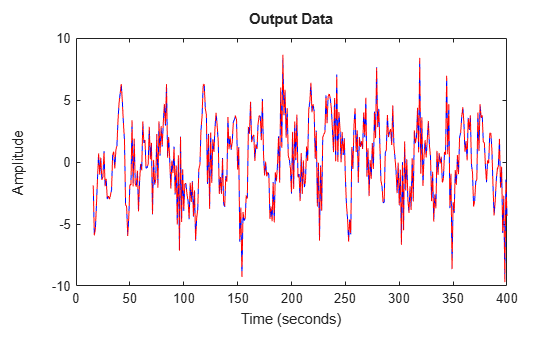`ysim2` is the same as `ysim`.

#### Continue Simulation of an Identified Model using Past States

Estimate the initial conditions for a simulation segment of an identified model by using the end states of the previous simulation segment.

Load data z1, which is an `iddata` object containing input and output data. Estimate a fifth-order linear model from the data.

```load iddata1 z1 plot(z1)```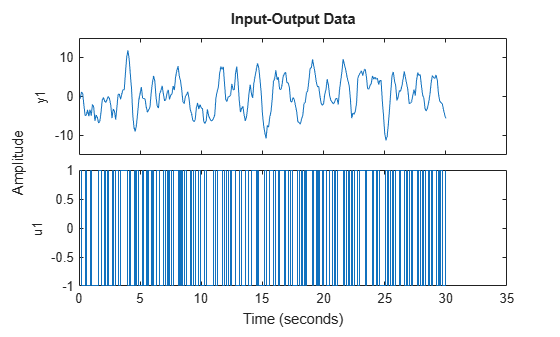`sys = n4sid(z1,5);`

Divide the data into two segments. One segment `z1past` represents data for a past simulation, and starts at rest. The second segment `z1next` represents data for a follow-on simulation.

```z1past = z1(1:150); z1next = z1(150:300);```

Simulate the response to the input data in `z1next` without specifying initial conditions.

```ynext_no_ic = sim(z1next,sys); plot(ynext_no_ic,z1next) legend title('Simulation Continuation Without Setting ICs')```Now determine the end states, `x0`, for the response to `z1past` input`.`

```[ypast,y_sd,xpast] = sim(z1past,sys); x0 = xpast(end,:)';```

Use the end states to specify the initial states for the follow-on response to `z1next`.

```opt = simOptions('InitialCondition',x0); ynext_ic = sim(z1next,sys,opt); figure plot(ynext_ic,z1next) legend title('Simulation Continuation with Sychronized ICs')```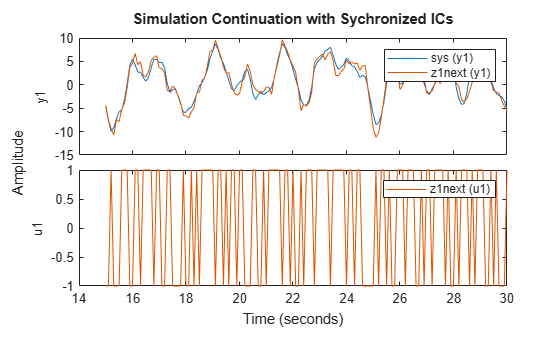### Estimate Initial Conditions for Simulating Hammerstein-Wiener and Nonlinear Grey-Box Models

When you are simulating a Hammerstein-Wiener or a nonlinear grey-box model and want to use initial conditions consistent with a measurement data set `z`, use `findstates`.

#### Simulate Hammerstein-Wiener Model in Simulink

Compare the simulated output of a Hammerstein-Wiener Model block to the measured output of a system. You improve the agreement between the measured and simulated responses by estimating initial state values.

```load twotankdata ```

Create an `iddata` object from the sample data. Set `'Tstart'` to 0 so that the data start time matches the Simulink start time 0f 0 s.

```z1 = iddata(y,u,0.2,'Tstart',0,'Name','Two tank system'); ```

Estimate a Hammerstein-Wiener model using the data.

```mw1 = nlhw(z1,[1 5 3],idPiecewiseLinear,idPiecewiseLinear); ```

You can now simulate the output of the estimated model in Simulink using the input data in `z1`. To do so, open a preconfigured Simulink model.

```model = 'ex_idnlhw_block'; open_system(model); ```The model uses the Iddata Source, Hammerstein-Wiener Model, and Scope blocks. The following block parameters have been preconfigured to specify the estimation data, estimated model, and initial conditions:

Block parameters of Iddata Source block:

• IDDATA Object - `z1`

Block parameters of Hammerstein-Wiener Model block:

• Model - `mw1`

• Initial conditions - `Zero` (default)

Run the simulation.

View the difference between measured output and model output by using the Scope block.

```simOut = sim(model); open_system([model '/Scope']) ```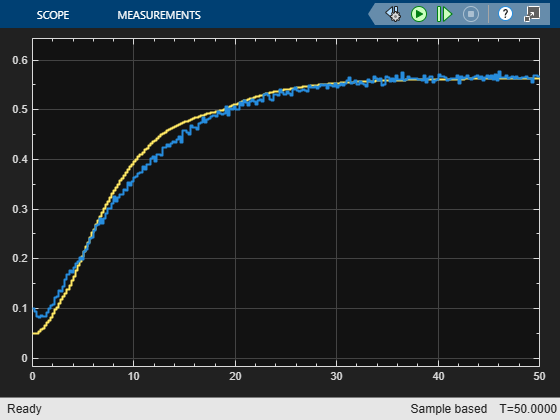To improve the agreement between the measured and simulated responses, estimate an initial state vector for the model from the estimation data `z1`, using `findstates`. Specify the maximum number of iterations for estimation as 100. Specify the prediction horizon as `Inf`, so that the algorithm computes the initial states that minimize the simulation error.

```opt = findstatesOptions; opt.SearchOptions.MaxIterations = 100; x0 = findstates(mw1,z1,Inf,opt); ```

Set the Initial conditions block parameter value of the Hammerstein-Wiener Model block to `State Values`. The default initial states are `x0`.

```set_param([model '/Hammerstein-Wiener Model'],'IC','State values'); ```

Run the simulation again, and view the difference between measured output and model output in the Scope block. The difference between the measured and simulated responses is now reduced.

```simOut = sim(model); ```### Estimate Initial Conditions for Simulating Nonlinear ARX Models

When you are simulating a nonlinear ARX model and want to use initial conditions consistent with a measurement data set `z`, you have a choice for which approach to take.

• You can use `findstates`. For nonlinear ARX models however, this approach has the cost of being computationally expensive if the data set is large.

`X0 = findstates(m,z,Inf);`
• You can break the data set into two portions. Use the first `nx` samples of the input/output data as past data, where `nx` is the largest regressor delay in the model. Then use `data2state` to compute the end state of the "past data." You are then able to simulate the model for the remaining `nx+1:end` input data with whatever simulation approach you choose. Both `compare` and the System Identification app use this approach automatically for nonlinear ARX models.

```nx = max(getDelayInfo(m)); % Find the largest regressor delay past_data = z1(1:nx); X0 = data2state(mw1,z1(1:nx));```

The following example illustrates the use of split-data technique for a nonlinear ARX model.

#### Estimate Initial Conditions for a Nonlinear ARX Model

Use two different techniques to estimate the initial conditions for a nonlinear ARX model. Simulate each alongside the measurement data, and compare with a simulation that initializes at rest.

Load the input/output data `z` and plot it. Use the first 1000 points of `z` to estimate a nonlinear ARX model.

```load twotankdata z = iddata(y,u,0.2); z1 = z(1:1000); plot(z1) title('Estimation data')```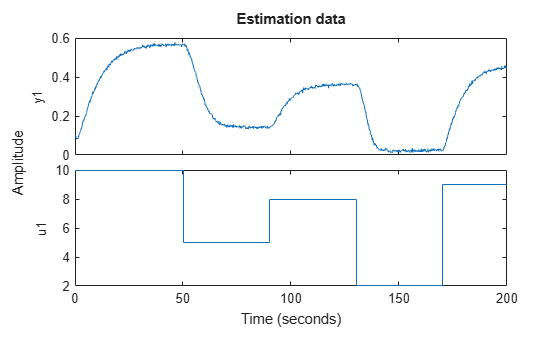`mdlnlarx = nlarx(z1,[5 1 3],idWaveletNetwork);`

Extract the first 250 points of z1 (50 seconds) as `z1sim` for comparison simulations. Simulate `mdlarx` without setting initial conditions, and plot the response alongside the measured output in `z1sim`.

```z1sim = z1(1:250); data_no_ic = sim(mdlnlarx,z1sim); plot(data_no_ic,z1sim) title('Nonlinear ARX Model with No Initial Condition Setting') grid on legend('location','se')```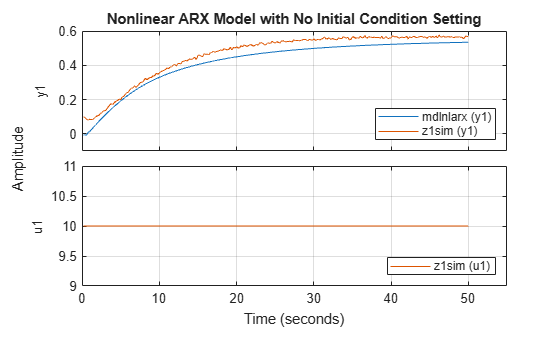Use `findstates` to estimate the initial conditions. In order to minimize the simulation error, specify `Inf` for the prediction horizon. Then set the `sim` option for `InitialCondition` to the `findstates` results. Plot the response alongside the measured output.

```x0fs = findstates(mdlnlarx,z1sim,Inf); opt = simOptions('InitialCondition',x0fs); data_fs = sim(mdlnlarx,z1sim,opt); figure plot(data_fs,z1sim) title('Nonlinear ARX Model with IC Estimation Using findstates') grid on legend('location','se')```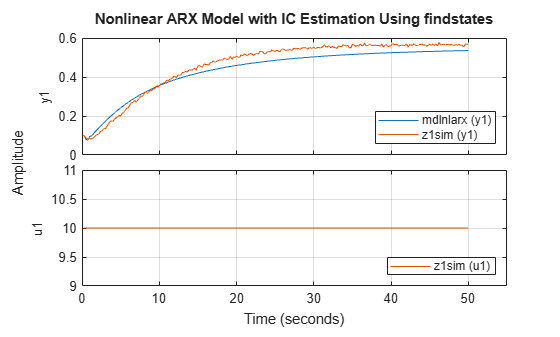The response and the measured output now start at roughly the same point.

Now use the `data2states` method to estimate the initial conditions. First, break the data set into two portions. Use the first `nx` samples of `z1sim` as "past data", where `nx` is the largest regressor delay in the model.

`nx = max(getDelayInfo(mdlnlarx))`
```nx = 5 ```
```z1past = z1sim(1:nx); z1sim2 = z1sim(nx+1:end);```

Use `data2state` to compute the end state of the "past data." Simulate the response using input starting at `z1sim(nx+1)`. Plot the response alongside the full `z1sim` measurement data so that you can compare with the `findstates` plot.

```x0d2s = data2state(mdlnlarx,z1past); opt = simOptions('InitialCondition',x0d2s); data_d2s = sim(mdlnlarx,z1sim2,opt); figure plot(data_d2s,z1sim) title('Nonlinear ARX Model with IC Estimation Using data2state') grid on legend('location','se')```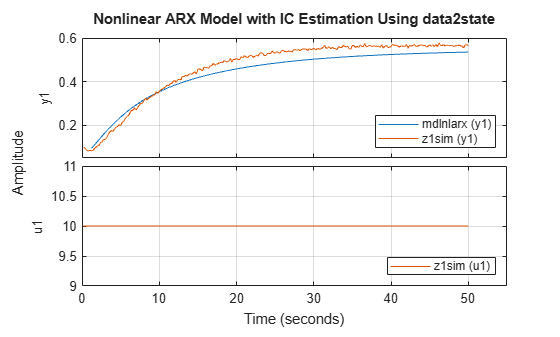If you executed each section separately, you may find that the `data2states` method completed more quickly than the `findstates` method.

Now compare the responses for all three initial-condition cases.

```plot(data_fs,data_d2s,data_no_ic) title('Nonlinear ARX model Responses for Three IC Approaches Compared') legend('location','se')```The responses for cases using `findstates` and `data2state` are virtually the same. The response for the case where the initial conditions were not set converges eventually, but not until after 30 seconds.